New Features in Maple 16: Differential GeometryWith over 250 commands, the DifferentialGeometry package allows sophisticated computations from basic jet calculus to the realm of the mathematics behind general relativity. In addition, 19 differential geometry lessons, from beginner to advanced level, and 6 tutorials illustrate the use of the package in applications.

In Maple 16, the DifferentialGeometry package introduces important new functionalities for working with abstractly defined differential forms, general relativity, and Lie algebras.

• You can now work with differential forms which are defined without reference to any underlying system of coordinates.
• The new MetricSearch assistant provides a simple method for searching the database of solutions to the Einstein equations.
• New commands for analyzing the geometric properties of spacetime are introduced.
• A new tutorial for differential geometry and general relativity has been added.
• Twenty-four new commands allow working with simple and semi-simple Lie algebras, including performing a complete analysis of the structure theory for any semi-simple Lie algebra.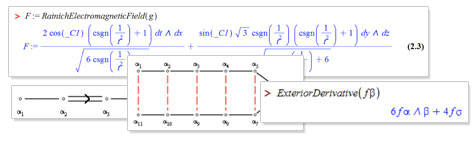Details

Abstractly defined differential forms

• The functionality of the command DGsetup has been extended to create a new computational environment for working with abstract differential forms, that is, differential forms which are defined without reference to any underlying system of coordinates. Structure equations for the abstract differential forms can be included in the new calling sequences for DGsetup. The capabilities of other commands in the DifferentialGeometry package, notably DGinfo, ExteriorDerivative, Hook and LieDerivative, have been extended to work with abstract differential forms. These capabilities extend those available in the difforms package.

The Maplet MetricSearch

• The Library sub-package contains a new command MetricSearch which provides a simple method for searching the DifferentialGeometry database of solutions to the Einstein equations.

General Relativity

• The Tensor sub-package contains 4 new commands for analyzing the geometric properties of space-times.
• The commands RainichConditions and RainichElectromagneticField can be used to determine if a given space-time is an electro-vac space-time and to find the electromagnetic field for that space-time.
• Line congruences play a distinguished role in the study of space-times and the properties of such congruences can now be easily calculated with the command CongruenceProperties.
• The signature of a metric can now be computed with QuadraticFormSignature. This procedure uses a simple algorithm which does not require eigenvalue computation and works well with the Maple assuming feature.

Structure Theory of Semi-simple Lie Algebras

• Twenty-four new commands have been added to the LieAlgebra package for working with simple (and semi-simple) Lie algebras. The DifferentialGeometry software now contains the commands for the complete analysis of the structure theory for any semi-simple Lie algebra (defined perhaps as a matrix algebra, a Lie algebra of symmetries of a differential equation, the isometries of a metric, the automorphisms of a Lie algebra, etc).
• The command SimpleLieAlgebraData can be used define the structure equations for any of the classical real simple Lie algebras,,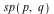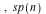. There are three companion procedures to SimpleLieAlgebraData. The procedure StandardRepresentation gives a basis for the standard representation of these Lie algebras as matrices. The procedure SimpleLieAlgebraProperties returns a table of properties for any one of these simple Lie algebras - these properties include Cartan subalgebra, root space decomposition, simple roots, positive roots, Cartan decomposition, etc. The command MatrixSubalgebra is used to create subalgebras of these classic matrix algebras.
• The command CartanSubalgebra finds a Cartan subalgebra for any given Lie algebra.
• The command RootSpaceDecomposition uses the Cartan subalgebra to produce a table which gives the root space decomposition of the Lie algebra. The roots can be extracted from the table with LieAlgebraRoots. The compact roots (purely imaginary) are found with CompactRoots. The root space decomposition is performed assuming that the Lie algebra is defined over the complex numbers. A real root space decomposition is obtained from RestrictedRootSpaceDecomposition.

• The command PositiveRoots will determine a set of positive roots from the root space decomposition. The command SimpleRoots finds the basis of simple roots from the positive roots.
• The Cartan matrix is found from the simple roots and the root space decomposition with CartanMatrix. The command CartanMatrixToStandardForm produces a re-ordering of the simple roots which transforms the Cartan matrix to standard form and identifies the complex type of the Lie algebra as "A", "B", "C", "D", "E", or "F". The command SatakeAssociate can be used to determine the real type of a given simple Lie algebra.
• The commands CartanDecomposition and CartanInvolution are available for semi-simple Lie algebras with a given matrix representation.
• The root space decomposition can be used to create gradings of a semi-simple Lie algebra or to find parabolic subalgebras.
• The signature of the Killing form can be computed with the KillingForm and QuadraticFormSignature commands.
• The Dynkin and Satake diagrams for a simple Lie algebra can be plotted with the commands DynkinDiagram and SatakeDiagram.

Query command

• The LieAlgebra Query command now supports keyword arguments "CartanDecomposition", "CartanInvolution", "CartanSubalgebra", "MatrixAlgebra", "NilRepresentation", "ParabolicSubalgebra", "RegularElement", "RootSpaceDecomposition", "SolvableRepresentation".

Examples

Example 1.

In this example we shall initialize the Lie algebra( the algebra of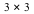trace-free matrices). For this algebra we then calculate [i] a Cartan subalgebra, [ii] the root space decomposition, [iii] the positive and simple roots, and [iv] the Cartan matrix.

 > with(DifferentialGeometry): with(LieAlgebras): with(Tensor): with(Tools):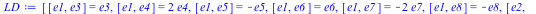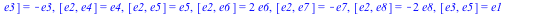Initialize this algebra.

 > DGsetup(LD);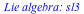Calculate a Cartan subalgebra.

 sl3 > CSA := CartanSubalgebra(sl3);Find the root space decomposition for this algebra.

 sl3 > RSD := RootSpaceDecomposition(CSA);Find the positive roots and the simple roots.

 sl3 > Rts:= LieAlgebraRoots(RSD);sl3 > PosRts := PositiveRoots(Rts, [<1,0>, <0, 1>]);sl3 > Delta := SimpleRoots(PosRts);Find the Cartan matrix.

 sl3 > CartanMatrix(Delta, RSD);Example 2.

A space-time is called an electro-vac spacetime if there exists an electromagnetic field which solves the Einstein-Maxwell field equations. The problem of deciding if a spacetime is electro-vac can be solved using the command RainichConditions and RainichElectromagneticField.

 M > DGsetup([t, x, y, z], M);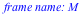M > g := evalDG(4/3*t^2* dx &t dx + t*(exp(-2*x)* dy &t dy + exp(2*x)*dz &t dz) - dt &t dt);Test to see if the Rainich conditions for this spacetime hold.

 M > RainichConditions(g);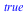We conclude the spacetime is an electro-vac spacetime. Here is the electro-magnetic field.

 M > F := RainichElectromagneticField(g);M > F := simplify(F) assuming t > 0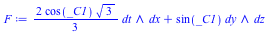We check that the Einstein equations are satisfied.

 M > T := EnergyMomentumTensor("Electromagnetic", g, F);M > E := EinsteinTensor(g);M > E &minus T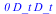We check that the Maxwell equations are satisfied.

 M > MatterFieldEquations("Electromagnetic", g, F);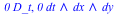Example 3.

The DifferentialGeometry package now supports extended functionality for working with abstract differential forms.

Create an abstract manifoldwith a function1-forms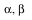and a 2-form.

 > DGsetup('[f = dgform(0) , alpha = dgform(1), beta = dgform(1), sigma = dgform(2)]', [], M);The command DGinfo gives the names of all scalars and forms which are defined.

 M > DGinfo("AbstractForms");Scalar products, wedge products and sums of abstract forms can be defined.

 M > omega := evalDG(2*alpha &wedge beta + 4*sigma);The command DGinfo can also be used to extract information about the form.

 M > DGinfo(omega, "ObjectFrame");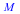M > DGinfo(omega, "FormDegree");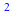M > DGinfo(omega, "CoefficientSet");M > DGinfo(omega , "CoefficientList", [sigma]);New forms can be defined on M.

 M > DGsetup(M, [delta = dgform(3)],[]);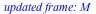M > DGinfo("AbstractForms");We can use the DGzip and GetComponents commands with abstract forms.

 M > Omega := evalDG([alpha &w beta, sigma]);M > zeta := DGzip([3, 5], Omega, "plus");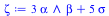M > GetComponents(zeta, Omega);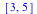We can take the exterior derivative of a form.

 M > rho:= ExteriorDerivative(alpha);The 2-form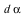has been added to list of defined forms and is now available for subsequent computations.

 M > DGinfo("AbstractForms");M > ExteriorDerivative(rho);Exterior derivatives of defined forms can be specified.

 M > DGsetup(M, [], [d(f) = f*alpha, d(beta) = 4*sigma + 5*alpha &wedge beta]);M > ExteriorDerivative(f*beta);How to Proceed: Pricing & Purchase Evaluate Upgrade Get Price Quote Buy Online